# 2020年高考高起点数学(文史财经类)押题卷一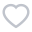488人已考
•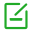卷面总分：150
•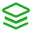试卷类型：模拟试题
•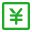测试费用： 免费
•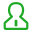关注人数：1566
•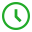作答时间：150分钟
•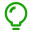解析：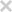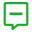试卷简介：
2020年高考高起点数学(文史财经类)押题卷一，本试卷是2020年高考数学(文)的全真模拟试题。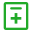试题类型：
• 单项选择题
• 填空题
• 解答题• 单项选择题
• 填空题
• 解答题
1

A.S∪T=S

B.S∪T=T

C.S∩T=S

D.S∩T=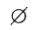2

A.-2

B.2

C.-1

D.2

3

A.10

B.8/5或136/5

C.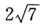D.32/5或8/5

4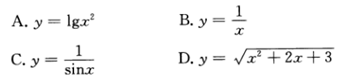A.

B.

C.

D.

5

A.关于x轴对称

B.关于原点对称

C.关于y轴对称

D.同一条曲线

6

A.(2，7)

B.(13，-7)

C.(2，-7)

D.(13，13)

7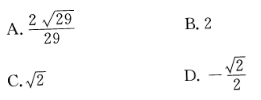A.

B.

C.

D.

8

A.（x-3）2+（y-2）2=4

B.（x+3）2+（y+2）2=4

C.（x-6）2+（y-4）2=4

D.（x+6）2+（y+4）2=4

9

A.b=3，ac=9

B.b=-3，ac=9

C.b=-3，ac=-9

D.b=3，ac=-9

10

A.1

B.-1

C.2

D.1/2